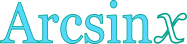Inverse sine calculator

This tool evaluates the inverse sine of a number: arcsin(x). The principal branch is evaluated, where the return values range between -π/2 and π/2. The domain of argument x is restricted to [-1,1].

 x = Result: arcsin(x) = deg radDefinitions

General

The inverse sine function, in modern notation written as arcsin(x), gives the angle θ, so that:

Because the values of sine function range between -1 and 1, the domain of argument x, in arcsin function, is restricted to the same range: [-1,1]. Also, due to the periodical nature of the sine function, there are many angles θ that can give the same sine value (i.e. θ+2π, θ+4π, etc.). As a result, it is impossible to define a single inverse function, unless the range of the return values is restricted, so that a one-to-one relationship between θ and sinθ can be established. Therefore, multiple branches of the arcsin function can be defined. Commonly, the desired range of θ values spans between -π/2 and π/2. The branch of arcsin, in that case, is called the principal branch.

Series

The arcsin function can be defined in a Taylor series form, like this:

The above series is valid for |x|≤1. From the expanded form of the series, it can be seen that the higher terms become insignificant, for values of x close to zero, resulting in the following quite useful approximation: